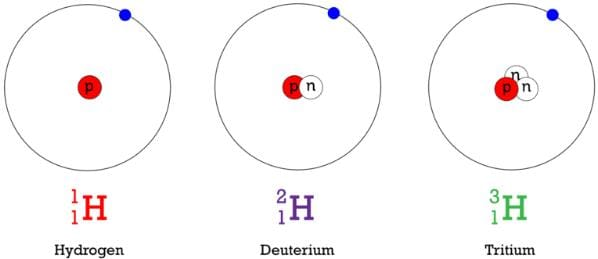NEET  >  Basic Terms Related Nucleus

# Basic Terms Related Nucleus Notes | Study Physics Class 12 - NEET

## Document Description: Basic Terms Related Nucleus for NEET 2022 is part of Physics Class 12 preparation. The notes and questions for Basic Terms Related Nucleus have been prepared according to the NEET exam syllabus. Information about Basic Terms Related Nucleus covers topics like and Basic Terms Related Nucleus Example, for NEET 2022 Exam. Find important definitions, questions, notes, meanings, examples, exercises and tests below for Basic Terms Related Nucleus.

Introduction of Basic Terms Related Nucleus in English is available as part of our Physics Class 12 for NEET & Basic Terms Related Nucleus in Hindi for Physics Class 12 course. Download more important topics related with notes, lectures and mock test series for NEET Exam by signing up for free. NEET: Basic Terms Related Nucleus Notes | Study Physics Class 12 - NEET
 1 Crore+ students have signed up on EduRev. Have you?

Nucleus:

The entire positive charge and nearly the entire mass of atom is concentrated in a very small space called the nucleus of an atom.

The nucleus consists of protons and neutrons. They are called nucleons.

Terms Related to Nucleus:

(i) Atomic Number: The number of protons in the nucleus of an atom of the element is called atomic number (Z) of the element.

(ii) Mass Number: The total number of protons and neutrons present inside the nucleus of an atom of the element is called mass number (A) of the element.

(iii) Nuclear Size: The radius of the nucleus R ∝ A1/3

⇒ R = Ro A1/3

where, Ro = 1.1 * 10-15 m is an empirical constant.

(iv) Nuclear Density: Nuclear density is independent of mass number and therefore same for all nuclei.

ρ = mass of nucleus / volume of nucleus ⇒ ρ = 3m / 4π R3o

where, m = average mass of a nucleon.

(v) Atomic Mass Unit: It is defined as 1 / 12th the mass of carbon nucleus.

It is abbreviated as amu and often denoted by u. Thus

1 amu = 1.992678 * 10-26 / 12 kg

= 1.6 * 10-27 kg = 931 Me V

Isotopes:

The atoms of an element having same atomic number but different mass numbers are called isotopes.

e.g., 1H1, 1H2, 1H3 are isotopes of hydrogen.Fig: Isotopes of hydrogen

Isobars:

The atoms of different elements having same mass numbers but different atomic numbers, are called isobars.

e.g., 1H3, 2He3 and 10Na22, 10Ne22 are isobars.

Isotones:

The atoms of different elements having different atomic numbers and different mass numbers but having same number of neutrons, are called isotones.

e.g., 1H3, 2He4 and 6C14, 8O16 are isobars.

Isomers:

Atoms having the same mass number and the same atomic number but different radioactive properties are called isomers.

Nuclear Force:

The force acting inside the nucleus or acting between nucleons is called nuclear force.

Nuclear forces are the strongest forces in nature.

• It is a very short range attractive force.
• It is non-central. non-conservative force.
• It is neither gravitational nor electrostatic force.
• It is independent of charge.
• It is 100 times that of electrostatic force and 1038 times that of gravitational force.

According to the Yukawa, the nuclear force acts between the nucleon due to continuous exchange of meson particles.

Mass Defect:

The difference between the sum of masses of all nucleons (M) mass of the nucleus (m) is called mass defect.

Mass Defect (Δm) = M – m = [Zmp + (A – Z)mn – mn]

Nuclear Binding Energy:

The minimum energy required to separate the nucleons up to an infinite distance from the nucleus, is called nuclear binding energy.

Nuclear binding energy per nucleon = Nuclear binding energy / Total number of nucleons

Binding energy, Eb = [Zmp + (A – Z) mn – mN]c2

Packing Fraction (P):

p = (Exact nuclear mass) – (Mass number) / Mass number

= M – A / M

The larger the value of packing friction. greater is the stability of the nucleus.

[The nuclei containing even number of protons and even number of neutrons are most stable.

The nuclei containing odd number of protons and odd number of neutrons are most unstable.]

The document Basic Terms Related Nucleus Notes | Study Physics Class 12 - NEET is a part of the NEET Course Physics Class 12.
All you need of NEET at this link: NEET

## Physics Class 12

157 videos|425 docs|213 tests
 Use Code STAYHOME200 and get INR 200 additional OFF

## Physics Class 12

157 videos|425 docs|213 tests

### How to Prepare for NEET

Read our guide to prepare for NEET which is created by Toppers & the best Teachers

Track your progress, build streaks, highlight & save important lessons and more!

,

,

,

,

,

,

,

,

,

,

,

,

,

,

,

,

,

,

,

,

,

;